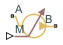# Flow Rate Source (TL)

Generate constant or time-varying mass flow rate or volumetric flow rate in thermal liquid network

Since R2023b

•Libraries:
Simscape / Foundation Library / Thermal Liquid / Sources

## Description

The Flow Rate Source (TL) block represents an ideal mechanical energy source in a thermal liquid network. The source can maintain the specified mass flow rate or volumetric flow rate regardless of the pressure differential. There is no flow resistance and no heat exchange with the environment. You specify the flow rate type by using the Flow rate type parameter.

The block icon changes depending on the values of the Source type and Flow rate type parameters.

Ports A and B represent the source inlet and outlet. The input physical signal at port M or V, depending on the flow rate type, specifies the flow rate. Alternatively, you can specify a fixed flow rate as a block parameter. A positive flow rate causes gas to flow from port A to port B.

The volumetric flow rate and mass flow rate are related through the expression

where:

• $\stackrel{˙}{m}$ is the mass flow rate from port A to port B.

• ρA and ρB are densities at ports A and B, respectively.

• $\stackrel{˙}{V}$ is the volumetric flow rate.

The energy balance at the source is a function of the energy flow rates through ports A and B and the work done on the fluid:

`${\varphi }_{A}+{\varphi }_{B}+{\varphi }_{work}=0,$`

where:

• ϕA is the energy flow rate into the source through port A.

• ϕB is the energy flow rate into the source through port B.

• ϕwork is the isentropic work done on the fluid.

The isentropic work term is

`${\varphi }_{work}=\frac{\stackrel{˙}{m}\left({p}_{B}-{p}_{A}\right)}{{\rho }_{avg}},$`

where:

• ϕwork is the isentropic work done on the thermal liquid.

• pA is the pressure at port A.

• pB is the pressure at port B.

• ρavg is the average liquid density,

`${\rho }_{avg}=\frac{{\rho }_{A}+{\rho }_{B}}{2}\text{.}$`

### Assumptions and Limitations

• There are no irreversible losses.

• There is no heat exchange with the environment.

## Ports

### Input

expand all

Input physical signal that specifies the mass flow rate through the source.

#### Dependencies

To enable this port, set the Source type parameter to `Controlled` and Flow rate type to `Mass flow rate`.

Input physical signal that specifies the volumetric flow rate through the source.

#### Dependencies

To enable this port, set the Source type parameter to `Controlled` and Flow rate type to `Volumetric flow rate`.

### Conserving

expand all

Thermal liquid conserving port. A positive flow rate causes the fluid to flow from port A to port B.

Thermal liquid conserving port. A positive flow rate causes the fluid to flow from port A to port B.

## Parameters

expand all

Select whether the flow rate generated by the source can change during simulation:

• `Controlled` — The flow rate is variable, controlled by an input physical signal. Selecting this option exposes the input port M or V, depending on the value of the Flow rate type parameter.

• `Constant` — The flow rate is constant during simulation, specified by a block parameter. Selecting this option enables the Mass flow rate or Volumetric flow rate parameter, depending on the value of the Flow rate type parameter.

Select the type of flow rate, `Mass flow rate` or `Volumetric flow rate`.

Desired mass flow rate of the fluid through the source.

#### Dependencies

To enable this parameter, set Source type to `Constant` and Flow rate type to `Mass flow rate`.

Desired volumetric flow rate of the fluid through the source.

#### Dependencies

To enable this parameter, set Source type to `Constant` and Flow rate type to `Volumetric flow rate`.

Select whether the source performs work on the fluid flow:

• `Isentropic` — The source performs isentropic work on the fluid to maintain the specified mass flow rate. Use this option to represent an idealized pump or compressor and properly account for the energy input and output, especially in closed-loop systems.

• `None` — The source performs no work on the flow, neither adding nor removing power, regardless of the mass flow rate produced by the source. Use this option to set up the desired flow condition upstream of the system, without affecting the temperature of the flow.

Area normal to flow path at the source inlet and outlet. The two cross-sectional areas are assumed identical.

## Version History

Introduced in R2023b

expand all